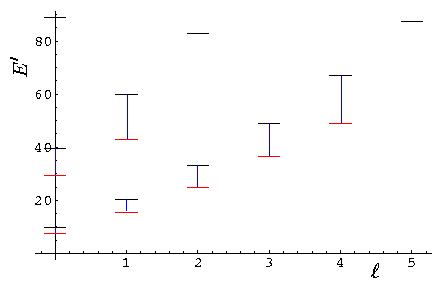## Square Wells p.16

### Finite Spherical Square Well Bound States

Outside of the well (i.e., r>a) we must have the exponentially decreasing "K" like spherical Bessel function (which we here call fl); Inside the well we must have the regular-at-the-origin spherical Bessel function jl: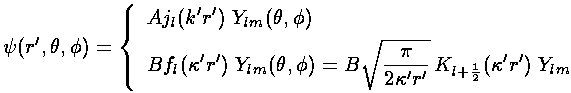where, as previously,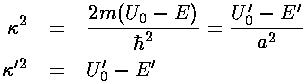These two solutions need to match up at the well boundary; we require bothandto be continuous at r=a. (The second derivative ofwill have a step at r=a to match the step in potential as required by Schrödinger's equation.) As before dividing the-match equation by the-match equation (i.e., matching the logarithmic derivative) eliminates the unknown normalization constants:Theequation is a nonlinear equation for the unknown k' given the size of the potential step: U0'. Clearly 0<k'<(U0')½. We examine the nature of the solutions by displaying the right hand side of the equation in blue and the left hand side in red. Solutions are where the two curves cross.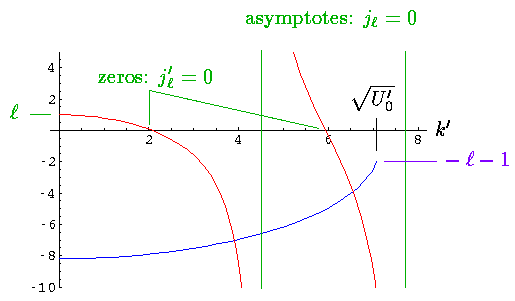The lhs (red) starts (k'=0) with y value l; it crosses the x-axis at the zeros of jl' and reaches ±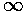asymptotically at the zeros of jl. The rhs (blue) is always negative since the fl(x) have negative slope; in fact, since for large x

fl(x)e-x

so fl'/fl should be approximately -1. Thus the rhs should start at nearly -(U0')½. It ends at k'=(U0')½ with a y value of -l-1. [The above plot is for l=1 and U0'=50 and shows two solutions. The wavefunctions are displayed below.] Clearly there will typically be an intersection for k' between a zero of jl' and a zero of jl. Unlike the lower dimensional square wells, there is not always a solution (i.e., a weak 3d well may have no bound states even though it's slightly attractive). We would just barely have a l=0 solution if the lhs reached -1 at k'=(U0')½. This will happen if U0' >2/4. [Mathematically the l=0 state is identical to the first excited state of the 1d square well...see the (R) differential equation and note the boundary condition that (R)=0 at r'=0.)

Once the magic value of k' is found, the following wavefunction has continuousand. The constant A is determined by normalizing the wavefunction.Here are some resulting wavefunction for U0'=50.Here is a display of the energy levels:In the low lying SHO states we would find much the same pattern, except we would have degeneracy between the second s state and the first d state and between the second p state and the first f state. Here we see "l-droop", the large l states in the SHO degenerate multiplet have drooped below the other states in the multiplet.

Below is a plot comparing the infinite square well energies with those of the U0'=50 system (each infinite square well energy [in black] is connected by a blue line to the equivalent finite square well energy [in red]).